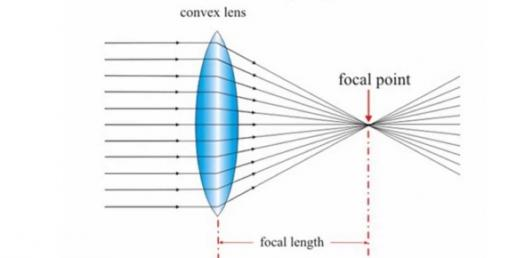# Quiz: What Do You Know About Thin Converging Lens? Trivia Questions

7 Questions | Attempts: 842
ShareSettingsWhat Do You Know About Thin Converging Lens? Thin lenses can be diverging or converging. The converging lenses are thicker in the middle than near the edges and as two focal points, located on the optical axis, a distance 'f' from the center of the lens on either side of the lens. Take up this test and get to see how much you know.

• 1.
A converging lens causes parallel light rays passing through it to __________ behind the lens.
• A.

Bend

• B.

Converge

• C.

Diverge

• D.

• 2.
Name two types of lens you learnt in this lesson. Write your answer in the form: _______, _______
• 3.
____________ is the horizontal line which passes symmetrically through the optical centre.
• 4.
The principal focus is also known as the ____________.
• 5.
When an object is placed exactly 2f away from a thin converging lens, the image formed is
• A.

Real, inverted, same size as object

• B.

Real, inverted, magnified

• C.

Virtual, upright, diminished

• D.

Virtual, upright, magnified

• 6.
When an object is placed a distance of less than f from a thin converging lens, an image is formed behind the object. What is a common device that makes use of this?
• A.

Camera

• B.

Magnifying glass

• C.

Photocopier

• D.

Spotlight

• 7.
When light rays passes through the optical centre of a lens, they will
• A.

Be refracted

• B.

Be dispersed

• C.

Pass through at an angle

• D.

Pass through undeviated

## Related TopicsBack to top
×

Wait!
Here's an interesting quiz for you.